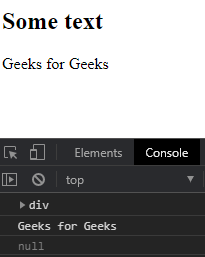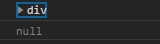# D3.js selection.node() Function

• Last Updated : 24 Nov, 2021

The selection.node() function in D3.js is used to return the first element in the selection. If the selection does not contain any elements then it returns null.

Syntax:

Hey geek! The constant emerging technologies in the world of web development always keeps the excitement for this subject through the roof. But before you tackle the big projects, we suggest you start by learning the basics. Kickstart your web development journey by learning JS concepts with our JavaScript Course. Now at it's lowest price ever!

`selection.node()`

Parameters: This function does not accept any parameters.

Return Values: This function returns the first element in the selection.

Example 1:

## HTML

 ```<``html` `lang``=``"en"``>` `<``head``>``    ``<``meta` `charset``=``"UTF-8"``>``    ``<``meta` `name``=``"viewport"` `path1tent``=``        ``"width=device-width,initial-scale=1.0"``>``    ``<``script` `src``=``"https://d3js.org/d3.v4.min.js"``>``    ````    ``<``script` `src``=``    ``"https://d3js.org/d3-selection.v1.min.js"``>``    ````` `<``body``>``    ``<``h2``>Some text``    ``<``div``>Geeks for Geeks ` `    ``<``script``>``        ``let selection = d3.selectAll("div")``        ``let div = selection.node();``        ``console.log(div)` `        ``// Printing innerHTML of the tag``        ``console.log(div.innerHTML)``        ``selection = d3.selectAll("h1")``        ` `        ``// Null is returned``        ``console.log(selection.node())``    ````` ``

Output:Example 2:

## HTML

 ```<``html` `lang``=``"en"``>` `<``head``>``    ``<``meta` `charset``=``"UTF-8"``>``    ``<``meta` `name``=``"viewport"` `path1tent``=``        ``"width=device-width,initial-scale=1.0"``>``    ``<``script` `src``=``"https://d3js.org/d3.v4.min.js"``>``    ````    ``<``script` `src``=``    ``"https://d3js.org/d3-selection.v1.min.js"``>``    ````` `<``body``>``    ``<``div``>Some text``    ``<``div``>Geeks for <``div``>geeks``    ````    ``<``div``>Geeks <``div``> for geeks``    ``<``div``>Some text` `    ``<``script``>``        ``let selection = d3.selectAll("div")``        ``console.log(selection.node())``        ``selection = d3.selectAll("h2")` `        ``// Null is returned``        ``console.log(selection.node())``    ````` ``

Output:My Personal Notes arrow_drop_up